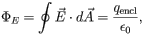# Problem: To understand the meaning of the variables in Gauss's law, and the conditions under which the law is applicable.Gauss's law is usually writtenwhere ε0 = 8.85 x 10-12 C2/(N • m2) is the permittivity of vacuum.How should the integral in Gauss's law be evaluated?a) around the perimeter of a closed loopb) over the surface bounded by a closed loopc) over a closed surface

###### FREE Expert Solution

a)

Around the perimeter of a closed loop, there is no field lines enclosed through the surface.

Gauss law cannot be evaluated around the perimeter of a closed-loop.

85% (403 ratings)###### Problem Details

To understand the meaning of the variables in Gauss's law, and the conditions under which the law is applicable.

Gauss's law is usually writtenwhere ε= 8.85 x 10-12 C2/(N • m2) is the permittivity of vacuum.

How should the integral in Gauss's law be evaluated?

a) around the perimeter of a closed loop

b) over the surface bounded by a closed loop

c) over a closed surface

Frequently Asked Questions

What scientific concept do you need to know in order to solve this problem?

Our tutors have indicated that to solve this problem you will need to apply the Gauss' Law concept. You can view video lessons to learn Gauss' Law. Or if you need more Gauss' Law practice, you can also practice Gauss' Law practice problems.